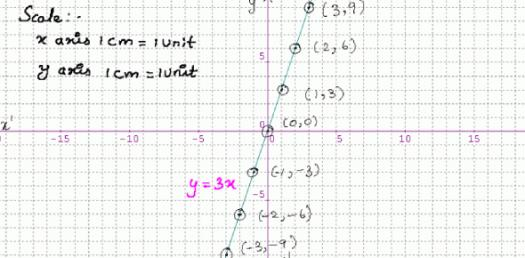# Algebra Understanding Direct Variation Quiz

Approved & Edited by ProProfs Editorial Team
The editorial team at ProProfs Quizzes consists of a select group of subject experts, trivia writers, and quiz masters who have authored over 10,000 quizzes taken by more than 100 million users. This team includes our in-house seasoned quiz moderators and subject matter experts. Our editorial experts, spread across the world, are rigorously trained using our comprehensive guidelines to ensure that you receive the highest quality quizzes.
| By Simonedeitch
S
Simonedeitch
Community Contributor
Quizzes Created: 6 | Total Attempts: 4,579
Questions: 8 | Attempts: 1,164Settings.

• 1.

• 2.

### Which of the following is NOT true about direct variation?

• A.

Direct Variation is y = k/x

• B.

Direct variation is y = kx

• C.

Direct variation always passes through the origin (0,0)

• D.

Direct variation has a constant of proportionality, k.

A. Direct Variation is y = k/x
Explanation
Direct Variation is y = k/x is not true because in direct variation, y is directly proportional to x, meaning that as x increases, y also increases. In the given equation, y is inversely proportional to x, as it decreases when x increases. Therefore, the equation y = k/x does not represent direct variation.

Rate this question:

• 3.

### Y is directly proportional to x. If y = 16 when x = 4, write the formula to represent this relation of y and x.

• A.

Y = 4x

• B.

Y = (1/4)x

• C.

Y = 12x

• D.

Y = 20x

A. Y = 4x
Explanation
The formula y = 4x represents the relation between y and x in this scenario. This means that y is directly proportional to x, and for every increase in x by 1 unit, y will increase by 4 units.

Rate this question:

• 4.

### Assume y varies directly as x.  If y = 20 and x  = 4, find y when x = 9.

• A.

Y = 9/5

• B.

Y = 180

• C.

Y = 14

• D.

Y = 45

D. Y = 45
Explanation
Since y varies directly as x, we can set up a proportion to solve for y. The proportion is y/x = k, where k is the constant of variation. We can find k by substituting the given values of y and x into the equation: 20/4 = k. Solving for k, we get k = 5. Now we can use the value of k to find y when x = 9: y/9 = 5. Solving for y, we get y = 45. Therefore, the answer is y = 45.

Rate this question:

• 5.

### If y = 15 when x = 2, then y = 10 when x = 8.  True or False?

• A.

True

• B.

False

B. False
Explanation
The statement is false because based on the given information, as x increases from 2 to 8, y should also increase. However, the statement claims that y decreases from 15 to 10, which contradicts the relationship established by the initial data. Therefore, the answer is false.

Rate this question:

• 6.

### The amount of money raised at a charity fundraiser is directly proportional to the number of attendees. The amount of money raised for 5 attendees was \$100. How much money will be raised for 60 attendees?

• A.

\$80

• B.

\$6,000

• C.

\$1,200

• D.

\$12,000

C. \$1,200
Explanation
The amount of money raised at a charity fundraiser is directly proportional to the number of attendees. This means that as the number of attendees increases, the amount of money raised will also increase. In this case, we are given that for 5 attendees, \$100 was raised. To find out how much money will be raised for 60 attendees, we can set up a proportion. If \$100 is raised for 5 attendees, then we can find out how much will be raised for 60 attendees by setting up the proportion: 5/100 = 60/x. Solving for x, we find that x is equal to \$1,200. Therefore, \$1,200 will be raised for 60 attendees.

Rate this question:

• 7.

### Which of the following is the correct equation for the following if y varies directly as x? x =3 and y = 4.

• A.

Y = (3/4)x

• B.

Y = (4/3)x

• C.

Y = 1x

• D.

Y = -1x

B. Y = (4/3)x
Explanation
If y varies directly as x, it means that y and x are directly proportional to each other. In other words, as x increases, y also increases in the same proportion. To find the equation that represents this relationship, we can use the formula y = kx, where k is the constant of variation. Given that x = 3 and y = 4, we can substitute these values into the equation and solve for k. By dividing both sides of the equation by 3, we find that k = 4/3. Therefore, the correct equation is y = (4/3)x.

Rate this question:

• 8.

### Katherine sells 75% of the cupcakes that she sells at her bakery.  Which term would correctly describe which part of the direct variation equation 75% would represent?

• A.

Y variable

• B.

X variable

• C.

Constant of variation

• D.

Product

C. Constant of variation
Explanation
In a direct variation equation, the constant of variation represents the relationship between the two variables. In this case, the constant of variation would represent the percentage of cupcakes sold by Katherine at her bakery. Since she sells 75% of the cupcakes, this percentage would be the constant of variation in the equation.

Rate this question:

Related TopicsBack to top